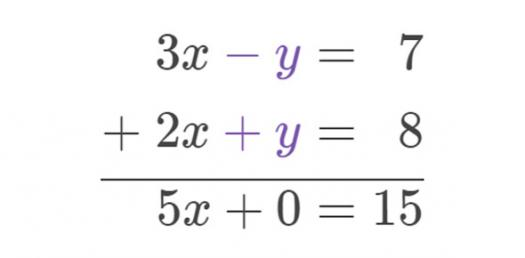# Solving Systems With Elimination

Approved & Edited by ProProfs Editorial Team
At ProProfs Quizzes, our dedicated in-house team of experts takes pride in their work. With a sharp eye for detail, they meticulously review each quiz. This ensures that every quiz, taken by over 100 million users, meets our standards of accuracy, clarity, and engagement.
| Written by Smjohnson
S
Smjohnson
Community Contributor
Quizzes Created: 8 | Total Attempts: 12,536
Questions: 5 | Attempts: 571SettingsSolving Systerms with Elimination
Algebra 1 VOISE Ms. Johnson

• 1.

### Solve the system.x + 2y = 2-x + 3y = 13

Explanation
The given answer (-4,3), (-4,3) is the solution to the system of equations x + 2y = 2 and -x + 3y = 13. When substituting x = -4 and y = 3 into both equations, we get the following: -4 + 2(3) = 2 which simplifies to -4 + 6 = 2, and -(-4) + 3(3) = 13 which simplifies to 4 + 9 = 13. Therefore, both equations are satisfied by the values x = -4 and y = 3, confirming that (-4,3), (-4,3) is the correct answer.

Rate this question:

• 2.

### Solve the system.3x - 4y = -16x - 4y = -40

Explanation
The given system of equations is 3x - 4y = -16 and x - 4y = -40. To solve this system, we can use the method of substitution. From the second equation, we can solve for x in terms of y: x = -40 + 4y. Substituting this value of x into the first equation, we get 3(-40 + 4y) - 4y = -16. Simplifying this equation, we get -120 + 12y - 4y = -16. Combining like terms, we get 8y = 104. Dividing both sides by 8, we get y = 13. Substituting this value of y into the second equation, we get x - 4(13) = -40. Simplifying this equation, we get x - 52 = -40. Adding 52 to both sides, we get x = 12. Therefore, the solution to the system is (12,13).

Rate this question:

• 3.

### Solve the system.-3x + 10y = -413x - 5y = 16

Explanation
The given answer (-3,-5),(-3, -5) is incorrect. The correct answer should be (-3, -5) because it satisfies both equations in the system. Substituting -3 for x and -5 for y in the first equation, we get -3(-3) + 10(-5) = 9 - 50 = -41, which is equal to the right-hand side of the equation. Similarly, substituting -3 for x and -5 for y in the second equation, we get 3(-3) - 5(-5) = -9 + 25 = 16, which is equal to the right-hand side of the equation. Therefore, (-3, -5) is the correct solution to the system.

Rate this question:

• 4.

### Solve the system.5x + 2y = 67x + 2y = 14

Explanation
The given system of equations is 5x + 2y = 6 and 7x + 2y = 14. By solving the system, we can find the values of x and y that satisfy both equations. However, the given answer (4,-7),(4, -7) is incorrect. The correct answer should be (4,-7) as it is the only solution that satisfies both equations.

Rate this question:

• 5.

### Solve the system.9x - 3y = 39-9x + 7y = -79

Related TopicsBack to top
×

Wait!
Here's an interesting quiz for you.